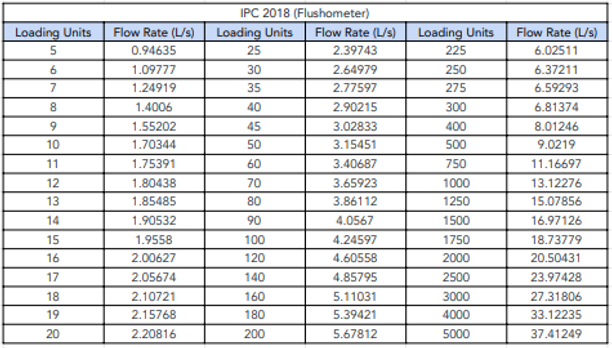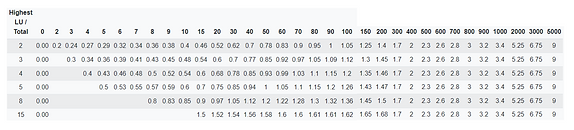# Probable Simultaneous Demand

The probable simultaneous demand (PSD) is determined by converting the loading units or full flow rate of all the fixtures that are downstream of the pipe into a flow rate.

The conversion from loading units or full flow rate to probable simultaneous demand is dependant on what conversion method the user chooses. We have the below options included in H2X:

DIN 1988-300:2012-05

The below equation is used with the variables noted for the different types of buildings.

Where the flow rate is below 0.2l/sec, the full flow rate is used.

Where the full flow rate is higher than 500 l/sec, a flow rate result will not be provided.ASNZS 3500.1 : 2018 - Dwellings

The below equation is used:

Flow Rate = 0.03n+0.4554√nInstitute of Plumbing Barrie's Book - DwellingsIPC 2018 - Flush TanksIPC 2018 - FlushometerUPC 2018 - Flush TanksUPC 2018 - FlushometerBS 6700 / IoP / CIBSE Guide GBS 806Note:

• In some cases, the flow rate of a single fixture will exceed the flow rate provided by the probable simultaneous demand calculation. For example, a birthing pool may have a flow rate of 1 L/sec but that will get diversified to < 1 L/sec. The pipe sizing, velocity, and pressure loss calculations in that pipe would need to be reviewed with modifications made.

• Heated water flow rates when using the DIN calculations do not allow for mixing of cold water to determine the flow rate. Therefore, the hot water flow rates will be slightly higher than necessary so modifications need to be made.

• Loading units / full flow rates of fixtures should always be reviewed and modified to suit your design

• Standards and guides that use graphs to display loading unit conversion to flow rates have been interpreted and may differ slightly from other interpretations

• Where a table does not stipulate the exact conversion for a loading unit to a flow rate, an estimate has been made between the below and above known conversions

# Continuous Flow

Continuous flow rates are added to the probable simultaneous demand flow rates to get the total flow rate on a system.

Flow Rate = Probable Simultaneous Demand + Continuous Flow Rates

# Cold Water Pipes Supplying Heated Water PlantWhere a cold water pipe supplies both the cold water to fixtures and also the heated water plant that supplies the heated water to the same fixtures, the flow rate will take the largest of the two flow rates.

This can be visualised in the below image where the flow rate on the cold water pipe is the same before and after the branch to the heated water plant.